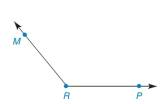Chapter 1.4, Problem 33E### Elementary Geometry for College St...

6th Edition
Daniel C. Alexander + 1 other
ISBN: 9781285195698

#### Solutions

Chapter
Section### Elementary Geometry for College St...

6th Edition
Daniel C. Alexander + 1 other
ISBN: 9781285195698
Textbook Problem
1 views

# In Exercises 33 to 40, use only a compass and a straightedge to perform the indicated constructions.Exercises 3 3 - 3 5 Given: Obtuse ∠ M R P Construct: With O A → as one side, an angle   ≅ ∠ M R P

To determine

To construct:

The angle congruent to MRP with OA as one side.

Explanation

Given:

The obtuse MRP is given.

The given figure is,

Figure (1)

Approach:

Use compass to make an arc that intersect both sides of MRP at point G and H as shown in figure (2).

Figure (2)

Make a ray OA as shown in figure (3)

Figure (3)

Make an arc to intersect OA at K of same radius as the previous arc as shown in figure (4)

### Still sussing out bartleby?

Check out a sample textbook solution.

See a sample solution

#### The Solution to Your Study Problems

Bartleby provides explanations to thousands of textbook problems written by our experts, many with advanced degrees!

Get Started

#### Find f. f(x) = 2x + 3ex

Single Variable Calculus: Early Transcendentals, Volume I

#### In Exercises 6372, evaluate the expression. 64. 4 + |4|

Applied Calculus for the Managerial, Life, and Social Sciences: A Brief Approach

#### limxx2+13x+2= a) 0 b) 1 c) 13 d) does not exist

Study Guide for Stewart's Single Variable Calculus: Early Transcendentals, 8th

#### True or false:

Study Guide for Stewart's Multivariable Calculus, 8th